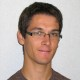# Sampling and Interpolation

This post will answer some of the questions posed at the end of the previous post, about what is a proper definition of sampling sequence.

Let us consider a function space$\mathcal{B} \subset L^2(\mathbb{R})$. We are interested in sequences$\{t_n\}$ where we can sample a function$f\in\mathcal{B}$. The first natural requirement is that the values of the function at$\{t_n\}$ uniquely define$f$ as an element of$\mathcal{B}$, meaning that no other function in$\mathcal{B}$ can have those same values at$\{t_n\}$. We will call such a sequence a uniqueness sequence.

Definition 1: Uniqueness Sequence

Given a function space$\mathcal{B} \subset L^2(\mathbb{R})$, we say that a sequence$\{t_n\}$ is a uniqueness sequence if there are no two different functions$f,g\in\mathcal{B}$ that agree in$\{t_n\}$.

A uniqueness sequence is not necessarily useful in practice because no continuity (stability) is required, meaning that arbitrarily small errors in the samples can correspond to arbitrarily large errors in the function sampled.

For this reason we impose a stability condition on the definition of sampling.

Definition 2: Sampling Sequence

Given a function space$\mathcal{B} \subset L^2(\mathbb{R})$, we say that a sequence$\{t_n\}$ is a sampling sequence if there is a positive constant$K$, such that the following inequalty holds for every$f\in\mathcal{B}$:$\int |f(t)|^2 dt \leq K\sum |f(t_n)|^2.$

We note that we are imposing that the operator that, given the values of$f$ at the sampling sequence, gives$f$ is continuous (bounded).

Another important definition is the one of Interpolation Sequence, it can be seen as a dual definition of the one of sampling sequence.

Definition 3: Interpolation Sequence

Given a function space$\mathcal{B} \subset L^2(\mathbb{R})$, we say that a sequence$\{t_n\}$ is a interpolation sequence if for every square summable sequence$\{a_n\} \in l_2$ there exists a function$f\in\mathcal{B}$ such that, for every$n$:$f(t_n)=a_n$.

Let us now particularise and consider that$\mathcal{B}$ is the space of bandlimited function defined in the previous post.

The Fourier Transform is a natural bijection between$\mathcal{B}$ and$L^2(-\pi,\pi)$. Suppose that$\{t_n\}$ is a uniqueness sequence.

We have that, for$g\in L^2(-\pi,\pi)$:$\hat{g}(t_n)=.$

Using the inversion formula one can check that$\hat{g}\in \mathcal{B}$ and that all the function in$\mathcal{B}$ are of this form. If$g$ is a non-zero function then$\hat{g}\in \mathcal{B}$ is a non-zero function so$\hat{g}(t_n)$ has to be non-zero for some$n$, then$$ is non-zero for some$n$, so$e^{it_n\cdot}$ is a complete set in$L^2(-\pi,\pi)$ (meaning that it’s span is dense in$L^2(-\pi,\pi)$). It is straight forward to prove the converse of this statement. This means that uniqueness sequences in$\mathcal{B}$ correspond to complete sets in$L^2(-\pi,\pi)$.

As we will see sampling sequences are tighly connected to Frames (in the same way uniqueness is connected to complete sets). A further discussion on the topic of Frames will be given on a future post.

1.Joel Moreira says: